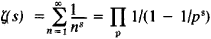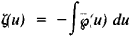Zeta Function

(redirected from Zeta functions)

zeta function

[′zād·ə ‚fəŋk·shən]
(mathematics)

Zeta Function

(1) An analytic function of a complex variable s = σ + it, defined for σ > 1 by the formulaL. Euler in 1737 introduced this function in mathematical analysis for real s, and in 1859 the German mathematician G. F. B. Riemann first studied it for complex 5, and therefore it is often called the Riemann zeta function. After the works of Euler (1748, 1749), P. L. Chebyshev (1848), and Riemann the profound relation between the properties of the zeta function and the properties of prime numbers was elucidated.

Euler calculated the values of ζ(2s) for any natural s. In particularFurther, he derived the identity (the Euler identity)where the product applies to all primes p = 2, 3, 5, . . . .

The zero distribution of the zeta function is of primary importance to the theory of primes. It is known that the zeta function has zeros at the points s = -2n, where n = 1, 2, . . . (these zeros are commonly called trivial zeros) and that all other (so-called nontrivial) zeros of the zeta function lie in the strip 0 < σ < 1, which is called the critical strip. Riemann proposed that all nontrivial zeros of the zeta function lie on the straight line σ = ½ . To date this hypothesis has been neither proven nor disproven. Important results on the zero distribution of the zeta function have been obtained by a new method in analytic number theory developed by the Soviet mathematician I. M. Vinogradov.

REFERENCES

Euler, L. Vvedenie v analiz beskonechnykh. 2nd ed., vol. 1. Moscow, 1961. (Translated from Latin.)
Wittacker, E. T., and G. Watson. Kurs sovremennogo analiza, 2nd ed., part 2. Moscow, 1963. (Translated from English.)
Titchmarsh, E. C. Dzeta-funktsiia Rimana. Moscow, 1947. (Translated from English.)
Ingham, A. E.Raspredelenie prostykh chisel Moscow-Leningrad, 1936. (Translated from English.)
Jahnke, E., and F. Emde. Tablitsy funktsii s formulami i krivymi. Moscow-Leningrad, 1948. (Translated from German.)
Prachar, K. Raspredelenie prostykh chisel. Moscow, 1967. (Translated from German.)
(2) In the theory of elliptic functions Weierstrass’ zeta function is encountered:where ζ(u) is Weierstrass’ elliptic function. This zeta function should not be confused with the Riemann zeta function.
References in periodicals archive ?
Let us begin by recalling the definition of absolute zeta functions from  and .
Multiple Zeta Functions, Multiple Polylogarithms and Their Special Values
Objective: The traditional (Riemann) approach to analytic number theory uses the zeros of zeta functions.
Euler products beyond the boundary for Selberg zeta functions .
They discuss such topics as extreme risk and fractal regularity in finance, zeta functions for infinite graphs and functional equations, lattice effects in the scaling limit of the two-dimensional self-avoiding walk, fractal oscillations near the domain boundary of radially symmetric solutions of p-Laplace equations, and applications of the contraction mapping principle.
The fine and coarse zeta functions, viewed as elements of the coarse incidence algebra, are equal.
The set, denoted by N, of the corresponding nonzero generalized zeta functions of complex order is defined by N := {[[xi].
Among the topics are partition functions and box-spline, the calculus of operator functions, toric Sasaki-Einstein geometry, automorphic representations, rigidity of polyhedral surfaces, number theory techniques in the theory of Lie groups and differential geometry, the flabby glass group of a finite cyclic group, Green's formula in Hall algebras and cluster algebras, zeta functions in combinatorics and number theory, and soliton hierarchies constructed from involutions.
Goldfeld is a world-class mathematician who has published over 50 papers and lectured internationally on a wide range of cryptographic topics and methods including applications of elliptic curves, quadratic fields, zeta functions, public-key cryptography, and group theoretic approaches to public-key cryptography.
Properly grouping the terms in expression for the coefficients we can use the Epstein zeta functions Z (A, 3) defined as
Chinen, Zeta functions for formal weight enumerators and the extremal property, Proc.
The proof of the main result depends on the description of the poles of the Igusa zeta function for non-degenerate polynomials , , , , and a theorem of Igusa that establishes a connection between the poles of local zeta functions and the asymptotic expansions of certain p-adic oscillatory integrals [6, Theorem 8.

Site: Follow: Share:
Open / Close